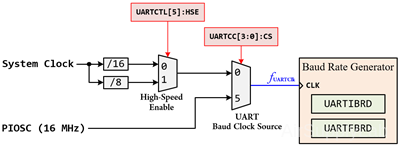# Lesson 15: Universal Asynchronous Receiver Transmitter (UART)

The TM4C123GH6PM and TM4C1294NCPDT controllers include eight UART ports (UART0 ~ UART7). Each of these ports features separate 16-by-8 transmit and receive FIFOs, a programmable baud rate generator, automatic generation and removal of the start, stop, and parity bits, line break generation, and detection, a choice of five to eight data bits, multiple parity types, and one or two stop bits, modem and flow control.

The UART is configured for transmitting and/or receiving via the TXE and RXE bits of the UART Control (UARTCTL) register. Before any control registers are programmed, the UART must be disabled by clearing the UARTEN bit in UARTCTL.

## Baud Rate Generation

The baud rate affects how fast the data can be sent. Baud rate is basically the frequency of the TX or RX pulses. The baud rate can be evaluated by the following formula:The UART clock source can be configured from the main system clock, the internal precision oscillator (PIOSC), or other clock selections.

The UART clock source for TM4C123G:

 UARTCC[3:0]CS UARTCTLHSE UART Clock Source 0 00 15 XThe baud rate generator allows speeds up to 5Mbps for regular speed (system clock divided by 16), or 10Mbps for high speed (system clock divided by 8)

The baud-rate divisor (BRD) is a 22-bit number consisting of a 16-bit integer and a 6-bit fractional part. The 16-bit integer is loaded through the UART Integer Baud-Rate Divisor (UARTIBRD) register and the 6-bit fractional part is loaded with the UART Fractional Baud-Rate Divisor (UARTFBRD) register. The baud-rate divisor has the following relationship to the UART:By default, the UART clock source is connected to Low-Speed mode (System Clock / 16). Therefore, the calculation of the baud rate divisor will be translated as follows:The UARTIBRD is the integer part of the BRD.

$$UARTIBRD = BR{D_{Integer}}\quad < = 65,535$$

The UARTFBRD can be calculated by taking the fractional part of the BRD, multiplying it by 64, and adding 0.5 to account for rounding errors.

$$UARTFBRD = Integer(BR{D_{Fraction}} \times 64 + 0.5)$$

For example, assume system clock frequency = 8 MHz, and the baud rate which we want the UART to be running at is 19200 bps. Therefore, the value of divisor is:1. Take 26 as the integer part and let it be BRDInteger. So, we have BRDInteger = 26.
2. Take 0.04167 as the decimal part and let it be BRDFraction. So, we have BRDFraction =  integer( 0.04167 * 64+ 0.5 ) = 3
3.  Save BRDInteger (= 26) to UARTIBRD register, and BRDFraction (= 3) to UARTFBRD register.

## Initialization and Configuration

To use the UART, the peripheral clock must be enabled by setting the appropriate bit in the RCGCUART register. In addition, the clock to the appropriate GPIO module must be enabled via the RCGCGPIO register in the System Control module.

Before to use UART peripheral, the following information must be decided and calculated:

1. Define the UART configuration for both devices:
• Baud rate: 1200, 2400, 4800, 9600, 19200, 38400, 57600, 115200 bps or higher rate
• Data length: 5, 6, 7, or 8 bit
• Stop bit: 1 or 2 bits
• Parity check mode: None, Odd, or Even
• Flow control mode: None, Software or Hardware flow control
• FIFOs mode: disable or enable FIFOs
2. Select the clock source (UARTCC[3:0]:CS) for UART module: from System Clock or PIOSC. If the clock source is from the system clock, the divider is either /16 (UARTCTL=0) or /8 (UARTCTL=1).
3. Calculate baud-rate divisor (BRD), then calculate BRDInteger and BRDFraction value
4. Find out which GPIO port and pins support UART peripheral, and its PMCn value in the GPIOPCTL register (Lesson 02: Verilog HDL)

To enable and initialize the UART, the following steps are necessary:

• Setup UART
1. Enable clock to UART Module using RCGCUART register, the allows time for clock stabilize
2. Disable UART by clearing UARTEN in UARTCTL register
3. Configure the baud rate to IBRD and FBRD registers
4. Configure data setup of the UART in  UARTLCRH register)
5. Configure the UART clock source in UARTCC register
6. Enable UART by setting UARTEN in UARTCTL register
• Setup GPIO pins for UART
1. Enable the clock to the appropriate GPIO module via the RCGCGPIO register
2. Configure the PMCn fields in the GPIOPCTL register to assign the UART signals to the appropriate pins
3. Set the GPIOAFSEL bits for the appropriate pins
4. There is no direction register (GPIODIR) for the UART pins because I/O pins are assigned automatically
5. Enable GPIO Pins in GPIODEN register